mscroggs.co.uk
mscroggs.co.uksubscribe

# Blog

2017-03-08
This post appeared in issue 05 of Chalkdust. I strongly recommend reading the rest of Chalkdust.
Take a long strip of paper. Fold it in half in the same direction a few times. Unfold it and look at the shape the edge of the paper makes. If you folded the paper $$n$$ times, then the edge will make an order $$n$$ dragon curve, so called because it faintly resembles a dragon. Each of the curves shown on the cover of issue 05 of Chalkdust is an order 10 dragon curve.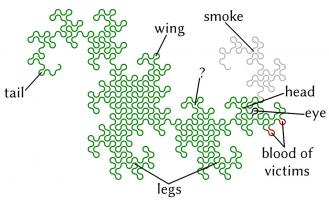Top: Folding a strip of paper in half four times leads to an order four dragon curve (after rounding the corners). Bottom: A level 10 dragon curve resembling a dragon.
The dragon curves on the cover show that it is possible to tile the entire plane with copies of dragon curves of the same order. If any readers are looking for an excellent way to tile a bathroom, I recommend getting some dragon curve-shaped tiles made.
An order $$n$$ dragon curve can be made by joining two order $$n-1$$ dragon curves with a 90° angle between their tails. Therefore, by taking the cover's tiling of the plane with order 10 dragon curves, we may join them into pairs to get a tiling with order 11 dragon curves. We could repeat this to get tilings with order 12, 13, and so on... If we were to repeat this ad infinitum we would arrive at the conclusion that an order $$\infty$$ dragon curve will cover the entire plane without crossing itself. In other words, an order $$\infty$$ dragon curve is a space-filling curve.
Like so many other interesting bits of recreational maths, dragon curves were popularised by Martin Gardner in one of his Mathematical Games columns in Scientific American. In this column, it was noted that the endpoints of dragon curves of different orders (all starting at the same point) lie on a logarithmic spiral. This can be seen in the diagram below.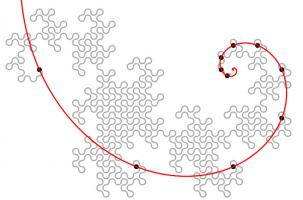The endpoints of dragon curves of order 1 to 10 with a logarithmic spiral passing through them.
Although many of their properties have been known for a long time and are well studied, dragon curves continue to appear in new and interesting places. At last year's Maths Jam conference, Paul Taylor gave a talk about my favourite surprise occurrence of a dragon.
Normally when we write numbers, we write them in base ten, with the digits in the number representing (from right to left) ones, tens, hundreds, thousands, etc. Many readers will be familiar with binary numbers (base two), where the powers of two are used in the place of powers of ten, so the digits represent ones, twos, fours, eights, etc.
In his talk, Paul suggested looking at numbers in base -1+i (where i is the square root of -1; you can find more adventures of i here) using the digits 0 and 1. From right to left, the columns of numbers in this base have values 1, -1+i, -2i, 2+2i, -4, etc. The first 11 numbers in this base are shown below.
 Number in base -1+i Complex number 0 0 1 1 10 -1+i 11 (-1+i)+(1)=i 100 -2i 101 (-2i)+(1)=1-2i 110 (-2i)+(-1+i)=-1-i 111 (-2i)+(-1+i)+(1)=-i 1000 2+2i 1001 (2+2i)+(1)=3+2i 1010 (2+2i)+(-1+i)=1+3i
Complex numbers are often drawn on an Argand diagram: the real part of the number is plotted on the horizontal axis and the imaginary part on the vertical axis. The diagram to the left shows the numbers of ten digits or less in base -1+i on an Argand diagram. The points form an order 10 dragon curve! In fact, plotting numbers of $$n$$ digits or less will draw an order $$n$$ dragon curve.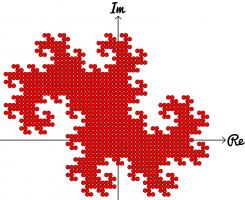Numbers in base -1+i of ten digits or less plotted on an Argand diagram.
Brilliantly, we may now use known properties of dragon curves to discover properties of base -1+i. A level $$\infty$$ dragon curve covers the entire plane without intersecting itself: therefore every Gaussian integer (a number of the form $$a+\text{i} b$$ where $$a$$ and $$b$$ are integers) has a unique representation in base -1+i. The endpoints of dragon curves lie on a logarithmic spiral: therefore numbers of the form $$(-1+\text{i})^n$$, where $$n$$ is an integer, lie on a logarithmic spiral in the complex plane.
If you'd like to play with some dragon curves, you can download the Python code used to make the pictures here.

### Similar posts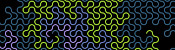Dragon curvesHarriss and other spirals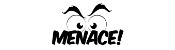MENACE at Manchester Science Festival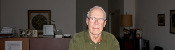The Mathematical Games of Martin Gardner

Comments in green were written by me. Comments in blue were not written by me.

Allowed HTML tags: <br> <a> <small> <b> <i> <s> <sup> <sub> <u> <spoiler> <ul> <ol> <li>
To prove you are not a spam bot, please type "noitauqe" backwards in the box below (case sensitive):
2016-09-06
 This is the fifth post in a series of posts about tube map folding.
After my talk at Electromagnetic Field 2014, I was sent a copy of MC Escher Kaleidocycles by Doris Schattschneider and Wallace Walker (thanks Bob!). A kaleidocycle is a bit like a 3D flexagon: it can be flexed to reveal different parts of itself.
In this blog post, I will tell you how to make a kaleidocycle from tube maps.

• 12 tube maps
• glue

### Making the modules

First, fold the cover of a tube map over. This will allow you to have the tube map (and not just its cover) on the faces of your shape.
With the side you want to see facing down, fold the map so that two opposite corners touch.
For this step, there is a choice of which two corners to connect: leading to a right-handed and a left-handed piece. You should make 6 of each type for your kaleidocycle.
Finally, fold the overhanding bits over to complete your module.
The folds you made when connecting opposite corners will need to fold both ways when you flex your shape, so it is worth folding them both ways a few times now before continuing.

### Putting it together

Once you have made 12 modules (with 6 of each handedness), you are ready to put the kaleidocycle together.
Take two tube maps of each handedness and tuck them together in a line. Each map is tucked into one of the opposite handedness.
The four triangles across the middle form a net of a tetrahedron. Complete the tetrahedron by putting the last tab into the first triangle. Glue these together.
Take two more tube maps of the opposite handedness to those at the top of the tetrahedron. Fit them into the two triangles poking out of the top of the tetrahedron to make a second tetrahedron.
Repeat this until you have five connected tetrahedra. Finally, connect the triangles poking out of the top and the bottom to make your kaleidocycle.Previous post in seriesTube map stellated rhombicuboctahedron
This is the fifth post in a series of posts about tube map folding.

### Similar posts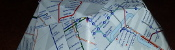Tube map Platonic solids, pt. 3Tube map stellated rhombicuboctahedronElectromagnetic Field talk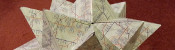Tube map Platonic solids, pt. 2

Comments in green were written by me. Comments in blue were not written by me.

Allowed HTML tags: <br> <a> <small> <b> <i> <s> <sup> <sub> <u> <spoiler> <ul> <ol> <li>
To prove you are not a spam bot, please type "r" then "a" then "t" then "i" then "o" in the box below (case sensitive):
2016-03-30
Take a piece of paper. Fold it in half in the same direction many times. Now unfold it. What pattern will the folds make?
I first found this question in one of Martin Gardner's books. At first, you might that the answer will be simple, but if you look at the shapes made for a few folds, you will see otherwise:
The curves formed are called dragon curves as they allegedly look like dragons with smoke rising from their nostrils. I'm not sure I see the resemblance:
As you increase the order of the curve (the number of times the paper was folded), the dragon curve squiggles across more of the plane, while never crossing itself. In fact, if the process was continued forever, an order infinity dragon curve would cover the whole plane, never crossing itself.
This is not the only way to cover a plane with dragon curves: the curves tessellate.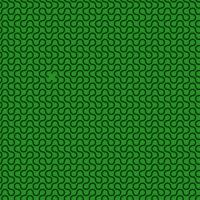When tiled, this picture demonstrates how dragon curves tessellate. For a demonstration, try obtaining infinite lives...
Dragon curves of different orders can also fit together:
To generate digital dragon curves, first notice that an order $$n$$ curve can be made from two order $$n-1$$ curves:
This can easily be seen to be true if you consider folding paper: If you fold a strip of paper in half once, then $$n-1$$ times, each half of the strip will have made an order $$n-1$$ dragon curve. But the whole strip has been folded $$n$$ times, so is an order $$n$$ dragon curve.
Because of this, higher order dragons can be thought of as lots of lower order dragons tiled together. An the infinite dragon curve is actually equivalent to tiling the plane with a infinite number of dragons.
If you would like to create your own dragon curves, you can download the Python code I used to draw them from GitHub. If you are more of a thinker, then you might like to ponder what difference it would make if the folds used to make the dragon were in different directions.

### Similar posts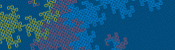Dragon curves IIPhD thesis, chapter 2Visualising MENACE's learningHarriss and other spirals

Comments in green were written by me. Comments in blue were not written by me.

Allowed HTML tags: <br> <a> <small> <b> <i> <s> <sup> <sub> <u> <spoiler> <ul> <ol> <li>
To prove you are not a spam bot, please type "r" then "a" then "t" then "i" then "o" in the box below (case sensitive):
2015-03-24
 This is the fourth post in a series of posts about tube map folding.
A while ago, I made this (a stellated rhombicuboctahedron):
Here are some hastily typed instructions for Matt Parker, who is making one at this month's Maths Jam. Other people are welcome to follow these instructions too.

• 48 tube maps
• glue

### Making a module

First, take a tube map and fold the cover over. This will ensure that your shape will have tube (map and not index) on the outside and you will have pages to tuck your tabs between later.
Now fold one corner diagonally across to another corner. It does not matter which diagonal you chose for the first piece but after this all following pieces must be the same as the first.
Now fold the overlapping bit back over the top.
Turn it over and fold this overlap over too.
You will need 48 of these and some glue.

### Putting it together

By slotting three or four of these modules together, you can make a pyramid with a triangle or square as its base.
A stellated rhombicuboctahedron is a rhombicuboctahedron with a pyramid, or stellation on each face. In other words, you now need to build a rhombicuboctahedron with the bases of pyramids like these. A rhombicuboctahedron looks like this:
More usefully, its net looks like this:
To build a stellated rhombicuboctahedron, make this net, but with each shape as the base of a pyramid. This is what it will look like 6/48 tube maps in:
If you make on of these, please tweet me a photo so I can see it!
Edit: Proof that these instructions can be followed: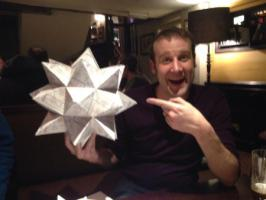Previous post in seriesTube map Platonic solids, pt. 3
This is the fourth post in a series of posts about tube map folding.
Next post in seriesTube map kaleidocycles

### Similar postsTube map Platonic solids, pt. 2Tube map kaleidocyclesTube map Platonic solids, pt. 3Electromagnetic Field talk

Comments in green were written by me. Comments in blue were not written by me.
I wish you'd make the final stellation of the rhombicuboctahedron! And show us! I know the shapes of the faces but have been stuck two years on the assembly!
Roberts, David

Allowed HTML tags: <br> <a> <small> <b> <i> <s> <sup> <sub> <u> <spoiler> <ul> <ol> <li>
To prove you are not a spam bot, please type "rotcev" backwards in the box below (case sensitive):
2015-03-03
This post explains how to make a trihexaflexagon with and images you like on the three faces.

### Making the template

To make a flexagon template with your images on, visit mscroggs.co.uk/flexagons. On this page, you will be able to choose three images (png, jp(e)g or gif) which will appear on the faces of your flexagon. Once you have created the template, save and print the image it gives you.
The template may fail to load if your images are too large; so if your template doesn't appear, resize your images and try again.

### Making the flexagon

First, cut out your printed tempate. For this example, I used plain blue, green and purple images.
Then fold and glue your template in half lengthways.
Next, fold diagonally across the blue diamond, being careful to line the fold up with the purple diamond. This will bring two parts of the purple picture together.
Do the same again with the blue diamond which has just been folded into view.
Fold the green triangle under the purple.
And finally tuck the white triangle under the purple triangle it is covering. This will bring the two white triangles into contact. Glue these white triangles together and you have made a flexagon.### Flexing the flexagon

Before flexing the flexagon, fold it in half through each pair of corners. This will get it ready to flex in the right places.
Now fold your flexagon into the following position.
Then open it out from the centre to reveal a different face.
This video shows how to flex a flexagon in more detail.

### Similar postsElectromagnetic Field talkAssorted christmathsDragon curves IITube map kaleidocycles

Comments in green were written by me. Comments in blue were not written by me.